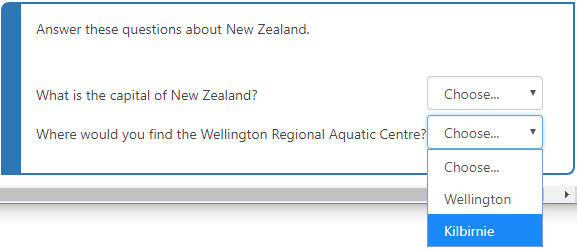• Totara 13
Examples of quiz question types
• 21 Jun 2022

# Examples of quiz question types

There are a range of question types available within the quiz module. The standard quiz question types are listed below with brief descriptions and examples.

Information on how to create these questions can be found on the Adding quiz questions page.

Please see administering question types for instructions on how to switch on/off certain question types at the site level.

Question typeDescriptionExample question

Multiple choice

Allows the selection of a single or multiple responses from a pre-defined list. The total grade must add up to 100%. If a single answer is correct, one answer must have the grade set to 100%.

MCQ question example - What kind of animal is a feline?True/False

A simple form of multiple choice question with just the two choices, True and False.

True/False example question - Totara Learn is open source?Matching

Matching questions ask the learner to match multiple question stems to multiple possible answers.

Matching question example - Match these countries with their capitals.Allows a response of one or a few words that is graded by comparing against various model answers, which may contain wildcards. You can use an asterisk (* ) to represent a wild card. For example, by typing in the answer as; hydrogen*oxygen the quiz would accept any of the following answers:

• hydrogen, oxygen
• hydrogen and oxygen
• hydrogen & oxygen
• hydrogen/ oxygen
• hydrogen + oxygen
• hydrogen; oxygen

Short answer example question - What elements make up water?Numerical

Allows a numerical response, possibly with units, that is graded by comparing against various model answers, possibly with tolerances.

Numerical question example - If I travel 100 miles in 2 hours, how fast am I traveling?Essay

An essay question is a free-response text area where learners can enter larger blocks of text in response to your question. These questions are not scored by the system and require manual grading.

Essay example question - Describe the key differences between ice cream and gelato.Calculated

Calculated questions are like numerical questions but with the numbers used selected randomly from a set when the quiz is taken.

Calculated question example - What is {a}+{b}?Calculated multi-choice

Calculated multi-choice questions are like multi-choice questions which choice elements can include formula results from numeric values that are selected randomly from a set when the quiz is taken.

Calculated multichoice question example - Calculate the area of a rectangle where l = {A}cm and h = {B}cm.Calculated simple

A simpler version of calculated questions which are like numerical questions but with the numbers used selected randomly from a set when the quiz is taken.

Calculated simple question example - . If I have {kittens} kittens and pet insurance costs £5 per animal - how much do I have to spend on pet insurance in total?Drag and drop into text

Missing words in the question text are filled in using drag and drop.

Drag and drop into text example question - This is an example of a ____. It starts with a ____ and ends with a ____.Drag and drop markers

Markers are dragged and dropped onto a background image.

Drag and drop marker example question - Label the colours of the rainbow.Drag and drop onto image

Images or text labels are dragged and dropped into drop zones on a background image.

Drag and drop onto image question example - Label the parts of the skeleton.These consist of a passage of text that has various answers embedded within it, including multiple choice, short answers, and numerical answers. The editor has been modified and allows you to test if your syntax is good. You can click Decode and verify the question text to test it.

Embedded answers (Cloze) example question - Match the following cities with the correct state.Like a Matching question, but created randomly from the short answer questions in a particular category.Select missing words

Missing words in the question text are filled in using dropdown menus.

Select missing words question example - Roses are ___ and violets are ___.Description

This is not a question and instead allows you to show some text (and possibly graphics) without requiring an answer. It is more of a label than a question type and may act as an introduction to a new section or may contain a resource (such as a video) that will be the basis for further questions.

-